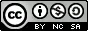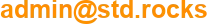# My Losing Lottery Numbers Script

I wanted to have a bash script which would be able to give lottery numbers. Unfortunately it never gives the good one...

## Code

```#! /bin/bash
# autolottery.sh version 1.0
# 2019.03.01  shebangthedolphins.net first version
#---------------------------------------------------
# this script automatically give (the wrong) lottery numbers. You can even set your own ones.
# it will gives 5 differents number from 1 to 49. And 1 complementary number from 1 to 10.
#---------------------------------------------------

#! /bin/sh

#Set variables
array=()
same="0"

#The script will ask if you have your own numbers to give
read -e -p "Give me manual numbers if you have some : " -a numbers #-a allows to put numbers into array

for manual_num in "\${numbers[@]}"
do
#"if" to put a 0 before numbers which are less than 10
if [ "\$manual_num" -lt 10 ]; then
manual_num=0\$manual_num
fi
array+=(\$manual_num) #add manual numbers to our array
done

#We need 5 numbers from 1 to 50
while [ "\${#array[*]}" -ne 5 ]
do
num=\$RANDOM
let 'num %= 50'
same="0"

for index in "\${array[@]}"
do
if [ "\$num" == "\$index" ] || [ "\$num" == "0" ] || [ 0"\$num" == "\$index" ] #if the number is equal to 0 or has already been proposed we exit the loop
then
same="1" #we put the flag same to 1
break #exit the loop
fi
done
#"if" to put a 0 before numbers which are less than 10
if [ "\$same" == "0" ] #if the number hasn't already been proposed
then
if [ "\$num" -lt 10 ]; then
num=0\$num
fi
fi
done

#allows to sort the numbers (https://stackoverflow.com/questions/7442417/how-to-sort-an-array-in-bash)
IFS=\$'\n' sorted=(\$(sort <<<"\${array[*]}"))

echo "Losing numbers are : "
for index in "\${sorted[@]}"
do
echo "\$index"
done

echo "Complementary number is : "
num="0"

while [ "\$num" == "0" ]
do
num=\$RANDOM
let 'num %= 11'
done

echo "\$num"

unset IFS

```